Mobile QR Code1. (Chung-Ang University, Seoul 06974, Korea )

Ultra-low power (ULP), Ultra-low voltage (ULV) operating SRAM, temperature effect inversion (TEI), system-on-chip

## I. INTRODUCTION

Internet of Things (IoT) devices are connected to a wireless network, collect data, upload data to the cloud, or exchange directly with other connected devices, ultimately providing analytics to help users make better choices. With the explosive increase in user needs for such analytics, continuous development of semiconductor process node scaling, high-performance low-power circuit and architecture designs, and wireless network evolutions have driven the growth of IoT devices. Furthermore, recent remarkable advances of AI technology are leading IoT devices to permeate almost every facet of our lives.

Among many technological advancements, in particular, low-power design is a core technology that enables the proliferation of IoT by the widespread use of IoT end-devices. IoT end-devices, located at the edge of the network and responsible for collecting data, are typically battery powered. Due to these characteristics, how long the device can perform sensing and simple data processing without charging the battery has become a key issue for realizing IoT end devices, thus various low-power techniques have been applied to IoT end devices. Dynamic voltage and frequency scaling (DVFS) and dynamic power management (DPM) are the most representative low-power techniques for system-on-chip (SoC) and have been actively applied to SoCs for early IoT end-device. However, as IoT end devices have been widely used, there is an increasing demand for ultra-low power (ULP) technology that can achieve more than the power saving levels achievable with the conventional DVFS and DPM [1-4].

Recently, ULP SoCs based on ultra-low voltage (ULV) operation have begun to establish themselves as SoCs that best meet the needs of state-of-the-art IoT end-devices.

More specifically, ULV operation SoC (ULV-SoC) is based on near-/sub-threshold voltage operation circuit, power consumption can be up to hundred times lower than the nominal voltage operation circuit. Of course, the significant power savings of ULV-SoC inevitably sacrifices huge performance degradation [5-10]. However, since IoT end devices have very low required performance (e.g., most IoT end devices operate at clock frequencies of tens or hundreds of MHz [11-14], reducing power consumption is a top priority, ULV-SoC becomes the best choice for IoT end devices.

In addition, recent research on the ULV-SoC reported that operating temperature vs. delay characteristic of the ULV-SoC is the opposite to that of conventional SoCs operating at nominal supply voltage, and ultimate ULP operation is achievable with this special feature [5, 15-22]. More precisely, in contrast to the general relationship that temperature and circuit operating speed are opposite, the ULV-SoC has a phenomenon that the speed increases as the temperature increases, which is called temperature effect inversion (TEI) phenomenon [15,18]. The advanced ULP techniques that exploit this TEI phenomenon to achieve the ultimate ULP operation has been intensively proposed, called the TEI-aware ULP (TEI-ULP) techniques, including the TEI-aware voltage scaling (TEI-VS [15, 18, 19], frequency up-scaling (TEI-FS) , power gating and frequency scaling (PGFS) , and body biasing (TEI-BB) [17,21]. Most recently, we have suggested that the TEI-ULP techniques may be limited in real SoC due to system interconnection problems and how to effectively solve it [5,22]. In that work, we have fabricated a real SoC in 28~nm FD-SOI technology, where the proposed method is applied, and experiments with the fabricated chip have demonstrated that TEI-ULP techniques can achieve the best ULP operation.

Although the TEI-ULP techniques have proven to have excellent power savings, SRAM, one of the essential IPs in SoC, have been excluded from the benefit. This is mainly due to the design difficulty of ULV-operating SRAM (ULV-SRAM), in that the ULV-SRAM is critically vulnerable to the process variation induced by the random doping fluctuations (RDF), degradation of on/off current ratio and imbalance between n-type MOSFET (nMOS) and p-type MOSFET (pMOS). As a result, there are no commercially available ULV-SRAMs from chip foundries, only nominal voltage-operated SRAMs, which prevent the TEI phenomenon from occurring in commercial chip SRAMs. In our previous chip presented in  for fully utilization of the TEI-ULP techniques, for example, we had no choice other than to use the SRAM provided and guaranteed by the chip foundry, thus SRAM had to be excluded from the application of TEI-ULP techniques. This power domain isolation of SRAM causes overhead due to an increase in the power pad and significant power overhead due to the SRAM.

In research field, although many studies have been conducted to propose new ULV-SRAM structures using additional transistors and assistive technologies to solve the process variation problem of ULV-SRAM [23-27], there has been no consideration of the TEI phenomenon and the application of TEI-ULP techniques in such studies. In other words, a question of whether availability of exploiting TEI-ULP techniques in such new ULV-SRAM has remained unknown. To resolve this issue and achieve chip-wide power savings, this paper studies on the existing ULV-SRAM structures and demonstrates that the TEI phenomenon is expressed in ULV-SRAM, and power saving can be achieved by applying the TEI-ULP techniques.

The remainder of this paper is organized as follows. In Section II, a preliminary review of the ULV-SRAM designs and the TEI-ULP techniques. Section III is dedicated to exploring the TEI design space with various ULV-SRAMs. In Section IV, the advanced TEI-ULP technique to address the stability issue of the ULV-SRAM, which makes it difficult to apply the existing TEI-ULP techniques to ULV-SRAM. Section V provides the intensive experimental work that verifies the efficacy of the proposed technique with three representative ULV-SRAM models and different stability requirements. Finally, Section V summarizes contents and concludes the paper.

## II. ANALYSIS OF EXISTING ULV-SRAM DESIGNS AND TEI-ULP TECHNIQUES

### 1. ULV-operating SRAM

Circuits operating in the ULV domain inevitably have problems of the degradation of on-current per off-current $\frac{I_{on}}{I_{off}}$ratio, nMOS/pMOS imbalance, and threshold voltage $V_{th}$ variation induced by RDF . To overcome these problems, intensive research have been studied since the early 2000s. With the advent of the IoT, the demand for ULPs has exploded, making this study more accelerated, and as a result, ULV circuits are now being commercialized.

To realize the ULV-SRAM, many studies have devised new topologies of the SRAM bitcell. More specifically, a bitcell structure composed of six transistors (6T) has been widely used in SRAM for traditional high-speed and high-density SoCs. Fig. 1(a) shows the traditional 6T SRAM bitcell structure, where BL and BLB are the bit line and bit line bar, respectively, WL is the wright line, and Q and QB are the cell storage nodes. In the 6T SRAM, read stability and write ability deteriorate as supply voltage $V_{DD}$ decreases.

To improve the read stability of ULV-SRAM, 8-transistor (8T) bitcell structure, shown in Fig. 1(b) has been proposed with additional skill of peripheral assists associated with the Buffer-Foot and $VV_{DD}$ . This bitcell structure focuses on the access transistor in the 6T bitcell structure. The access transistor in the 6T bitcell is used for both write and read operations, and the size of access transistor required for stable operation of write or read operation is opposite. At the nominal voltage operation, the margin of the size of the access transistor for write or read is sufficient, but at the ULV operation, the margin is drastically reduced. Therefore, the size of the access transistor for stable operation of write and read cannot be derived in the ULV operation. To address this, the 8T bitcell structure uses the access transistor only for write operation, and adds a new line called read buffer line (cf, RBL in Fig. 1(b)). Additionally, the 8T bitcell structure can reduce the leakage current of the inaccessible row and the on-current of the accessed row through the read buffer.

##### Fig. 1. Schematics of the bit cell structures for the ULV-SRAM: (a) 6T; (b) 8T; (c) 10T; (d) 12T.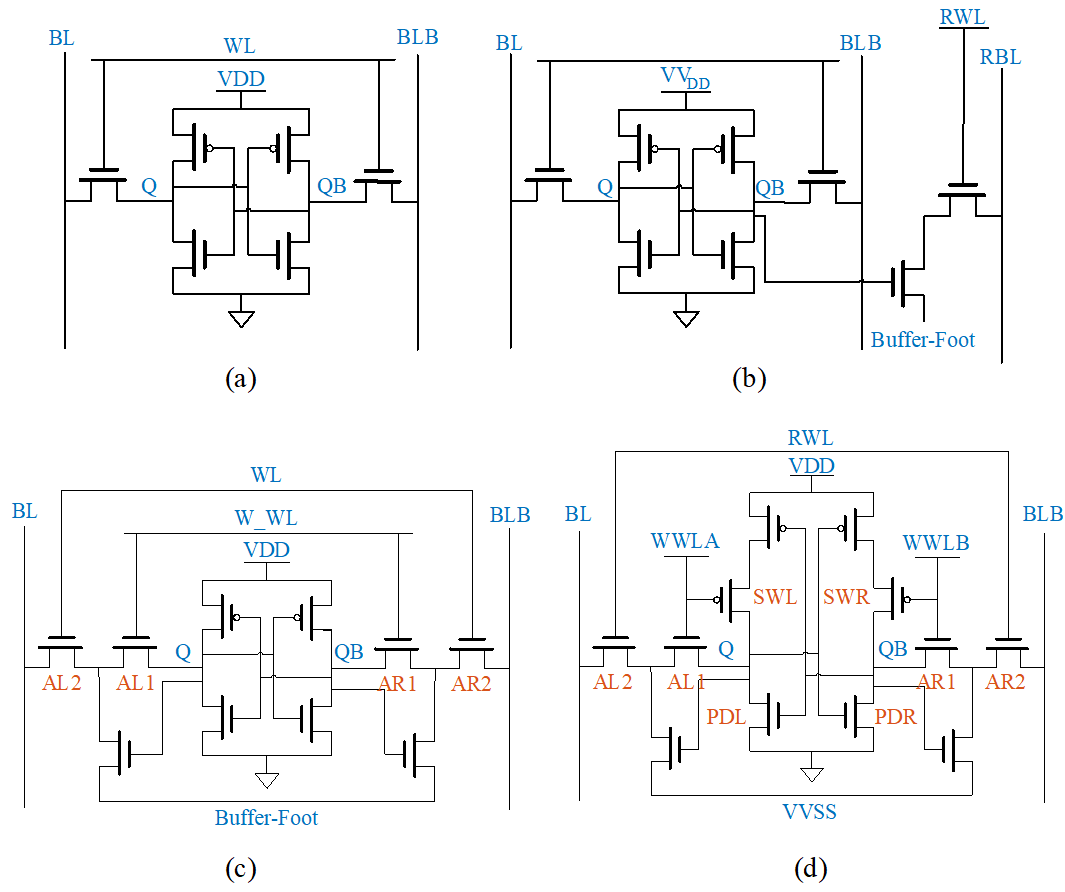The 8T bitcell structure provides a lower operating voltage than the 6T bitcell structure, but due to half-select disturbance, there is critical limitation to using the bit-interleaving structure  that modern SRAMs typically use to reduce the soft-error rate contribution of multi-bit errors . To tackle this limitation, a 10-transistor (10T) bitcell structure has been presented, which separates traditional WL into WL and \textit{W\_WL} by adding a read buffer to the 8T bit cell, as seen in Fig. 1(c). These WL and \textit{W\_WL} are shared by cells in rows and columns, respectively.

Because only \textit{W\_WL} of the selected cell in a write operation is raised, the half-select problem can be mitigated. In addition, the cell storage nodes Q and QB are decoupled form the bit lines during a read operation to improve read margin. Also, the 10T bitcell uses a VGND node that rises to $V_{DD}$ in the hold/write operation and is forced to zero in the read operation to reduce bit line leakage current.

However, at the 10T, the series access transistors (cf. AL1,2 and AR1,2 in Fig. 1(c)) introduced to easily solve the half-select problem can degrade the SRAM write ability. To improve the write ability, a 12-transistor (12T) bitcell structure has been proposed . As shown in Fig. 1(d), data-aware column-based word lines WWLA and WWLB are introduced, which change according to write data, enhancing the write ability. More precisely, for writing 0'', WWLA goes up to $V_{DD}$, and SWL is cut off. Hence, node Q is easily discharged by PDL. And vice versa, same principle is applied to WWLB and node QB during the write 1'' operation. These features reduce the impact of contention between pull-up pMOS and pass-gate nMOS, which improves the write ability as well as read stability and attenuated half-select disturbance.

Finally, the proposed bitcell structure for ULV-SRAM increases the size cost while guaranteeing stability compared to the 6T bitcell. For example, 8T, 10T, and 12T bitcells are 1.3X, 2.1X, and 2.13X larger, respectively, than conventional 6T bitcells . All three ULV-SRAM (except 6T) cells are well-known structures representing ULV-SRAM. However, TEI-ULP techniques have never been applied to these ULV-SRAMs. In order to expand the design space of TEI-ULP techniques, TEI-ULP techniques are briefly described, and the availability of the techniques in the existing ULV-SRAM and other considerations for applying TEI-ULP to SRAM are discussed in this paper. Designing an optimized SRAM structure for TEI-ULP techniques may be an optimized approach to achieving the full potential of such techniques, but we conduct this study as a more general way to apply TEI-ULP techniques with a more comprehensive approach, taking into account the characteristics of embedded SRAMs (each SRAM is utilized in different goals and operating conditions).

### 2. TEI-ULP Techniques

In VLSI circuit, the delay of a logic gate $\tau _{D}$ is directly affected by $I_{on}$, $\tau _{D}\propto \frac{1}{I_{on}}$. As a temperature-dependent function, $I_{on}$ can be expressed as :

##### (1)  (2)
$$$I_{o n} \propto \begin{cases}\mu(T) \cdot\left(V_{g s}-V_{t h}(T)\right)^{\beta} & : \text { if } V_{g s}>V_{t h}, \\ \mu(T) \cdot e^{\frac{V_{g s}-V_{t h}(T)}{S(T)}} & : \text { otherwise }\end{cases}$$$

where T is temperature; $V_{gs}$ is the gate-source voltage; S denotes the sub-threshold swing coefficient; $\mu$ is the carrier mobility; and $\beta$ is the velocity saturation effect factor. S, $\mu$, and $V_{th}$ are temperature-dependent device parameters, whereby $V_{th}$ and $\mu$ decrease while S increases as T rises. In (1), when the transistor operates in the super-threshold voltage regime, $I_{on}$ is mainly affected by mobility $\mu$. In a consequence, $I_{on}$ decreases with rising T. Therefore, the worst case corner of the conventional MOSFET transistors operating at super-threshold voltage occurs at the highest T in the operating temperature range. On the other hand, in (2), when the transistor operates in the ULV regime, $V_{th}$ mainly affect to $I_{on}$, and $\mu$ has some moderating effect in the opposite direction. Therefore, as T increases, $I_{on}$ becomes larger. In other words, $\tau _{D}$ of the ULV circuit decreases with increasing T, and its the worst case corner happens at the lowest operating T. This unique characteristics of the ULV circuit is called TEI (temperature effect inversion).

To go beyond the theoretical interpretation of the TEI phenomenon and to study how the TEI-ULP techniques achieve power savings in state-of-the-art semiconductor technology, we first performed simulations using a FO4 inverter chain based on the 28 nm FD-SOI technology node. Fig. 2 shows the simulation result of $\tau _{D}$ vs. T, and as expected, the delay decreases with rising T. Plus, it is observed that the smaller $V_{DD}$, the more clearly the TEI phenomenon occurs.

Owing to these characteristics, when the temperature of the circuit is higher than the lowest operating temperature (i.e., temperature of the worst case corner), the speed can be maintained while lowering $V_{DD}$, which results in significant amount of power savings in the circuit. To analyze this in more detail, first, power can be expressed in terms of $\mathrm{V}_{DD}$:

##### (3)
$P_{dynamic}=\alpha \cdot C\cdot V_{DD}^{2}\cdot f,\\ P_{static}=V_{DD}\cdot I_{off}$

where $P_{dynamic}$ and $P_{static}$ are dynamic power and static power consumption of the total power $P_{total}$, respectively; $\alpha$ is activity factor; C is capacitance of the circuit; and $f$ is the operating frequency. If the supply is reduced to the lowest $\mathrm{V}_{DD}$while maintaining the target f owing to the TEI phenomenon, both $P_{dynamic}$ and $P_{static}$ must be reduced.

This low power technique can be applied to the FO4 inverter chain in Fig. 2, which is detailed in Fig. 3. The operating temperature of the target FO4 inverter is $-40$$\mathrm{℃} to 125\mathrm{℃} with 0.6 V supply, thus the worst case corner speed is determined at -40$$\mathrm{℃}$. When T becomes $-2$$\mathrm{℃}, the circuit speed of 0.58 V operation becomes the same as that of the 0.6 V operation circuit, so at higher temperatures than -2$$\mathrm{℃}$, the clock frequency of the circuit can still be maintained even if 0.6 V is lowered to 0.58 V. Similarly, if $T\geq 32\mathrm{℃},\,\,V_{DD}$ can be lowered from 0.6 V to 0.56 V, and $V_{DD}$ can be further lowered as T becomes higher as shown in Fig. 3. This power saving mechanism is one of the representative TEI-ULP techniques, called TEI-VS [15, 18, 19].

##### Fig. 2. TEI phenomenon in the FO4 inverter chain based on 28~nm FDSOI technology.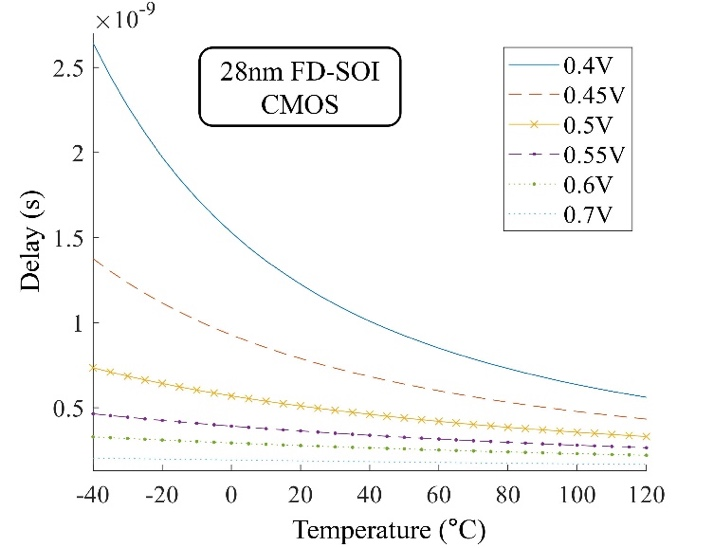##### Fig. 3. Example of TEI-VS applied to the FO4 inverter chain.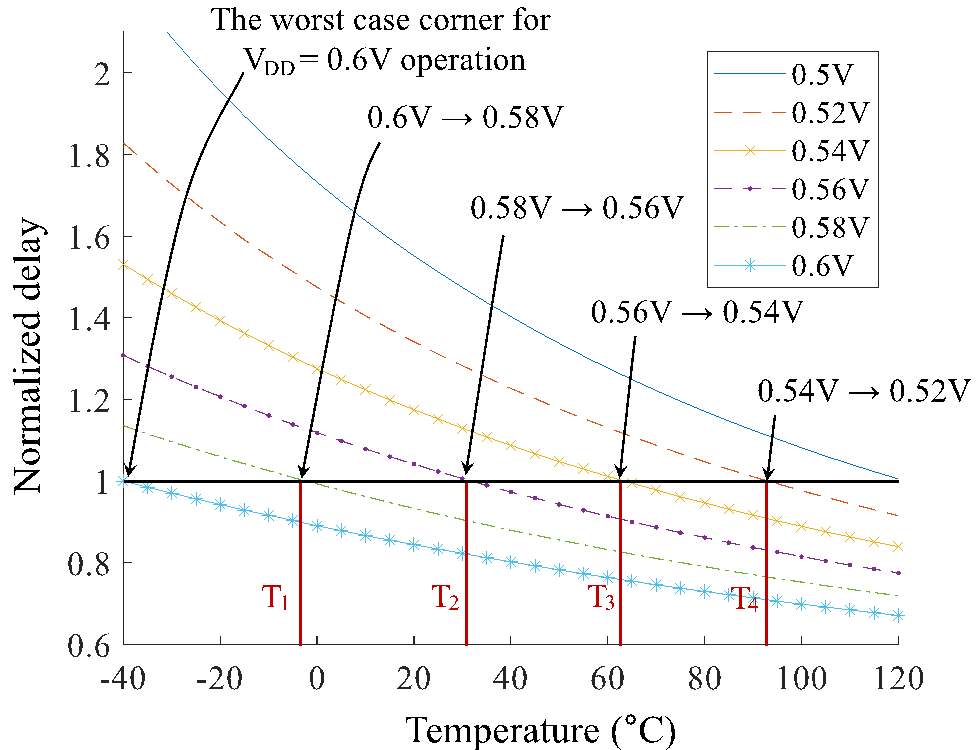## III. EXPLORATION AND ANALYSIS OF THE TEI PHENOMENON IN ULV-SRAM

TEI-VS shows excellent power saving effect in ULV circuit, but its application to ULV-SRAM has not been attempted yet. This may be because i) (from an industrial point of view) the use of nominal voltage-operated SRAM provided by the chip foundry is recommended when making SoCs, and ii) (from an academic point of view) there is no study of TEI phenomenon of ULV-SRAM. If it turns out that TEI-VS can be applied to ULV-SRAM to achieve great power consumption reduction, it will further spur the development of ULV-SRAM, which is evolving as in Section II.1. Motivated by this, we intend to study the TEI phenomenon of ULV-SRAM for the first time.

To study the TEI phenomenon in ULV-SRAM, we first studied the difference between the TEI phenomenon in nMOS and pMOS. Unlike the previous studies on the TEI phenomenon, which discussed the TEI phenomenon of the entire gate logic without separately distinguishing nMOS and pMOS as shown in Fig. 2, SRAM is so sensitive to the nMOS/pMOS imbalance. Therefore, in ULV-SRAM, the TEI phenomenon for each nMOS and pMOS must be considered separately. For this reason, we performed simulations of $I_{on}$ for each nMOS and pMOS according to T and $V_{DD}$ changes, each of which is shown in Fig. 4(a) and (b), respectively. Both cases in the figure, $I_{on}$'s are normalized by the worst case corner of $I_{on}$ that occurs when $V_{DD}=0.6$V and $T=-40\mathrm{℃}$. At the worst case corner, $I_{on}$ of nMOS, pMOS are $83.1\mu A$, $61\mu A$, respectively. From the simulation results, we can observe new interesting facts:

##### Fig. 4. Simulation results of $I_{on}$ of (a) nMOS; (b) pMOS vs. T, for varying $V_{DD}$.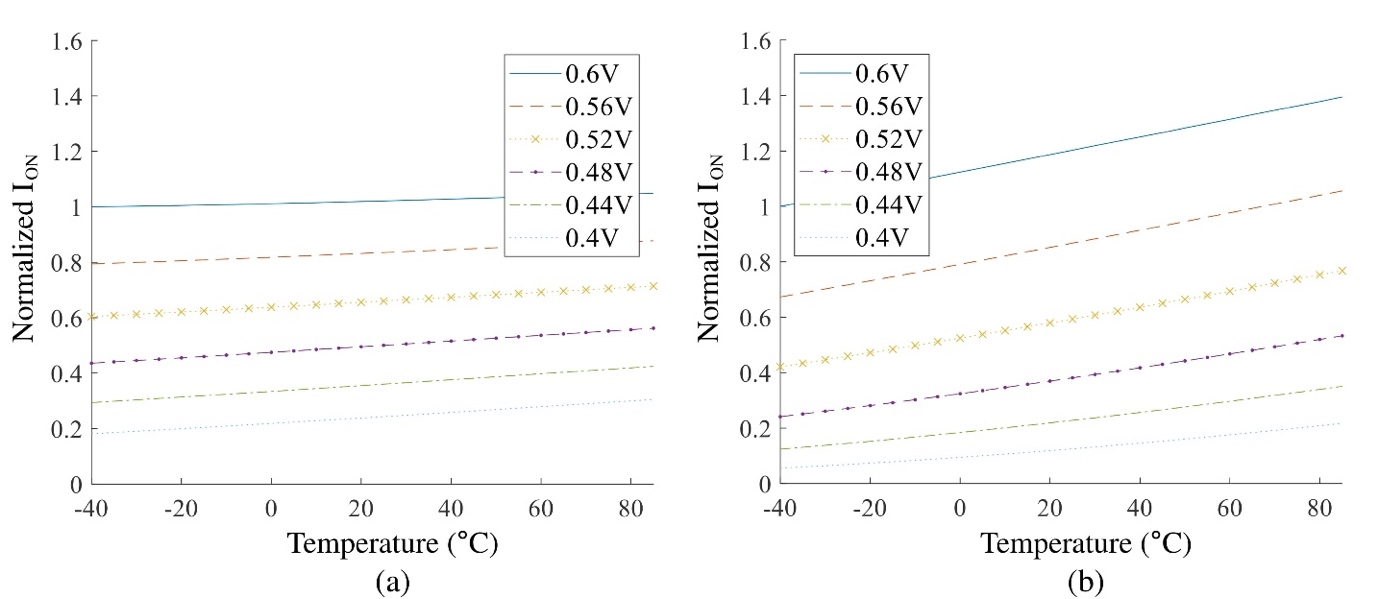·In the ULV regime ($T<0.6$V) the smaller the $V_{DD}$, the more clearly the TEI phenomenon in the case of nMOS, whereas in the case of pMOS, the TEI phenomenon clearly occurs in all $V_{DD}$'s.

·At the same $V_{DD}$, the amount of $I_{on}$ change according to T change is larger in pMOS than in nMOS.

Taking these observations into account, we perform stability and timing analysis of ULV-SRAM in the following subsection. In these analysis, we set up the analysis environment as the target SRAM for use in a previously developed System-on-Chip (SoC) platform (called TEI-inspired SoC Platform, TIP) operating at $-40$$\mathrm{℃}$ to 85$\mathrm{℃}$ and equipped with a DC-DC converter with 10 mV voltage adjustable resolution.

### 1. Stability Analysis

In this paper, research on SRAM that can have value as a one-chip solution in combination with TIP using TEI-VS is conducted, and such SRAM is defined as TEI-SRAM. One of the most critical issues in the TEI-SRAM is whether it is stable due to lowering the supply voltage. Generally, the lower the supply voltage, the lower the stability of the SRAM . In addition, operating SRAM at high temperatures also threatens stability. This is due to the relative intensity (i.e., lowered by the increase in temperature of the ULV aforementioned in section 2.1) is strongly correlated with stability . Therefore, we should first check the stability of the ULV-SRAM model in the operating environment of TIP.

Main benchmark of the stability on SRAM is static noise margin (SNM) of bit-cell . SNM is the maximum amount of noise that guarantees the flip-well of each cell during write operations and retains data during read/hold operations. As aforementioned, read stability and write ability is major concern for ULV-SRAMs. To investigate the stability of the four different designs of ULV-SRAM, i.e., 6T, 8T, 10T and 12T, designed using Cadence tool with the 28 nm FDSOI PDK, we measured the read SNM (RSNM) and write SNM (WSNM)  of the model, respectively.

Fig. 5 and 6 show the measured RSNM and WSNM of each ULV-SRAM model with respect to temperature. First of all, the experimental results clearly show that the higher the temperature and the lower the voltage, the lower the SNM of each cell. Looking more closely, from Fig. 5, it can be confirmed that the RSNM of the 6T model is significantly lower than that of the other models, while the 8T, 10T, and 12T models are much higher, ensuring high read stability.

In Fig. 6, it can be seen that the 10T model has better write ability than the 12T model, which is different from the known results. Analyzing the reason, when $V_{DD}$ becomes 0.5 V in 28 nm FDSOI technology, the strength of pMOS is very low compared to nMOS so that the beta ratio is almost 27, which significantly diminishes merits of write ability in 12T model. Therefore, considering the area overhead induced by using additional transistors, it can be concluded that 8T and 10T models are better choices for ULV-SRAM based on 28 nm FDSOI technology. When the operating voltage and temperature are generally set, the margin in Fig. 5 and 6 may be sufficient to operate ULV-SRAM (8T, 10T, and 12T models) reliably, but considering the tendencies that the margin decreases at high temperature and low supply voltage, careful control is required to apply TEI-techniques, especially TEI-VS, to SRAM. Additionally, due to extremely low RSNM, We will progress our work with 8T, 10T, and 12T model.

##### Fig. 5. RSNM of (a) 6T; (b) 8T, 10T; (c) 12T models in operating ranges.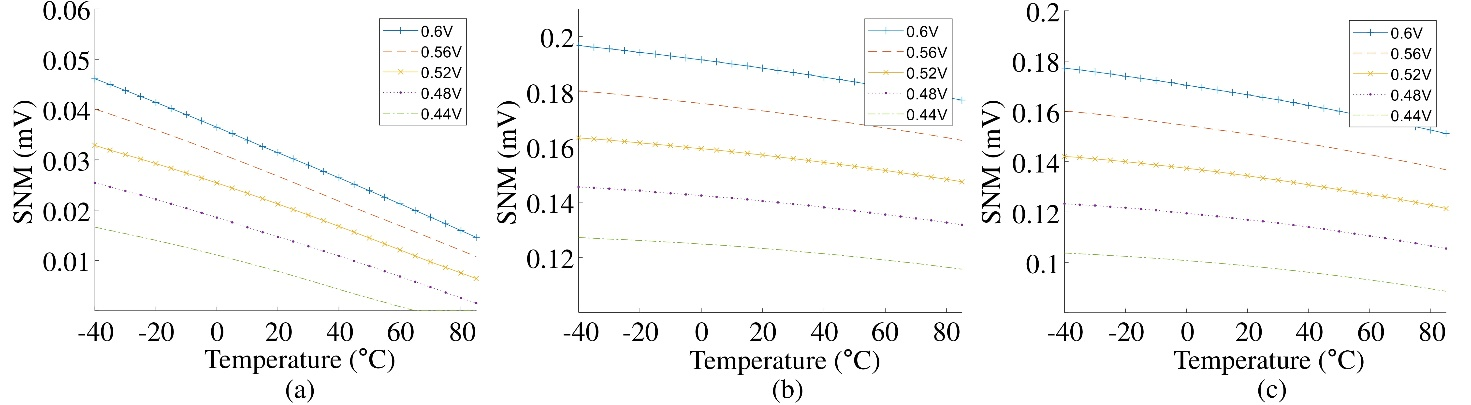##### Fig. 6. WSNM of (a) 6T; (b) 8T, 10T; (c) 12T models in operating ranges.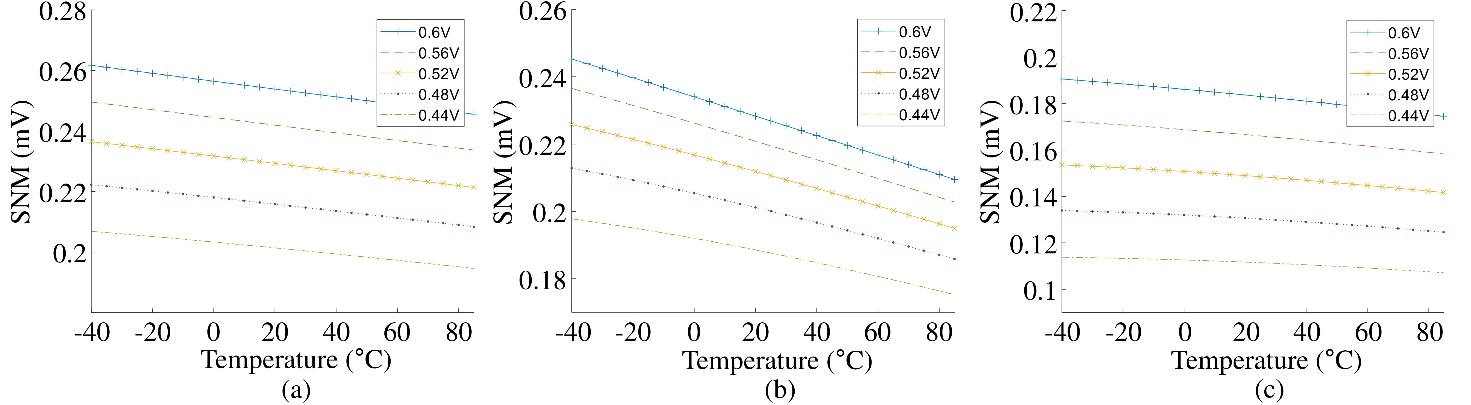### 2. Timing Analysis

For TEI-SRAM, it is necessary to analyze whether each SRAM model exhibits the TEI phenomenon and, if so, how much influence it has. To this end, we conducted a timing analysis using the designs of each SRAM model used in the previous subsection. At this time, it was found that the 6T model was not suitable for TEI-SRAM through stability analysis, so the 6T model was excluded from the analysis. Timing analysis was carried out by specifically measuring the write access time $\tau _{WA}$ and read time $\tau _{R}$ of the relevant models. First, $\tau _{WA}$ is the duration between charging WL 50% and the moment the storage node reaches 90% of supply voltage while writing '1' (which reaches 10% when writing "0")  measured. And the read time was measured with a simple latch-type voltage sense amplifier  that does not participate in digital logic.

Meanwhile, $\tau _{R}$ was measured from the time WL reached 50% of its maximum value, i.e., the supply voltage, to the time required for the output signal of the sense amplifier.

Fig. 7 and 8 show $\tau _{WA}$ and $\tau _{R}$ of the three different SRAM models over a wide range of temperature values. In the figures, the delay results are normalized to the delay at $V_{DD}=~ 0.7\mathrm{V}$and T = 85$^{\circ}$C. As seen in the figure, $\tau _{WA}$ and $\tau _{R}$decrease as rising T. More precisely, setting the supply voltage to 0.44 V causes the $\tau _{WA}$ at 0$^{\circ}$C to drop to almost half that value at 80$^{\circ}$C on the 8T model. And both $\tau _{WA}$and $\tau _{R}$show similar trends in the 10T and 12T models. Through this, we can figure out that TEI-VS can be applied to any types of SRAM model. That is, the 8T, 10T, and 12T models are all suitable for TEI-SRAM in terms of timing, and the supply voltage of the SRAM can be reduced at a higher temperature while maintaining the target operating speed.

##### Fig. 7. Write access time ($T_{WA}$) of (a) 8T; (b) 10T; (c) 12T model with respect to temperatures.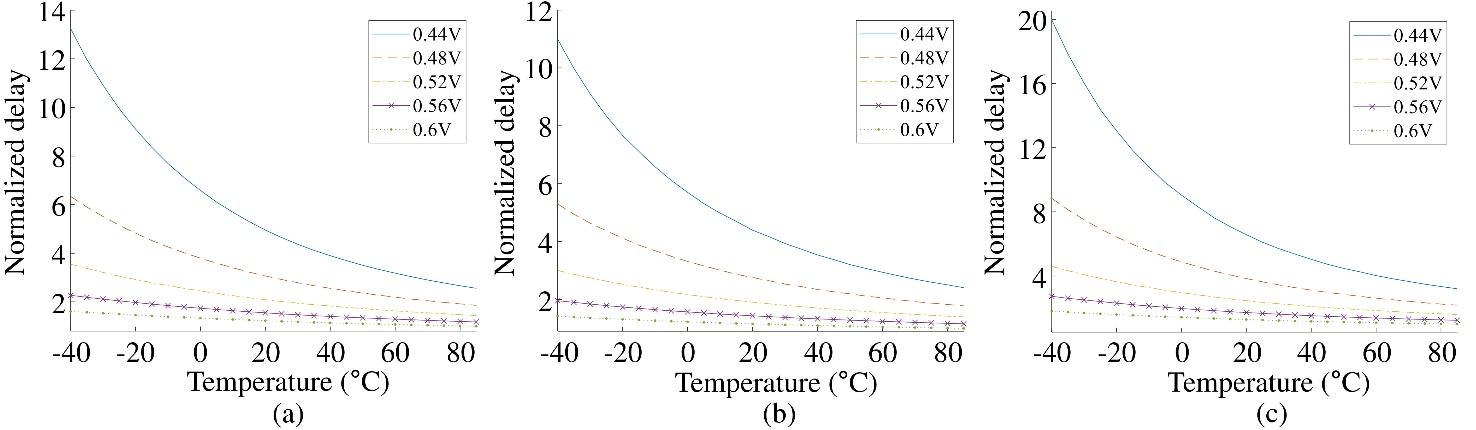##### Fig. 8. Read access time ($T_{R}$) of (a) 8T; (b) 10T; (c) 12T model with respect to temperatures.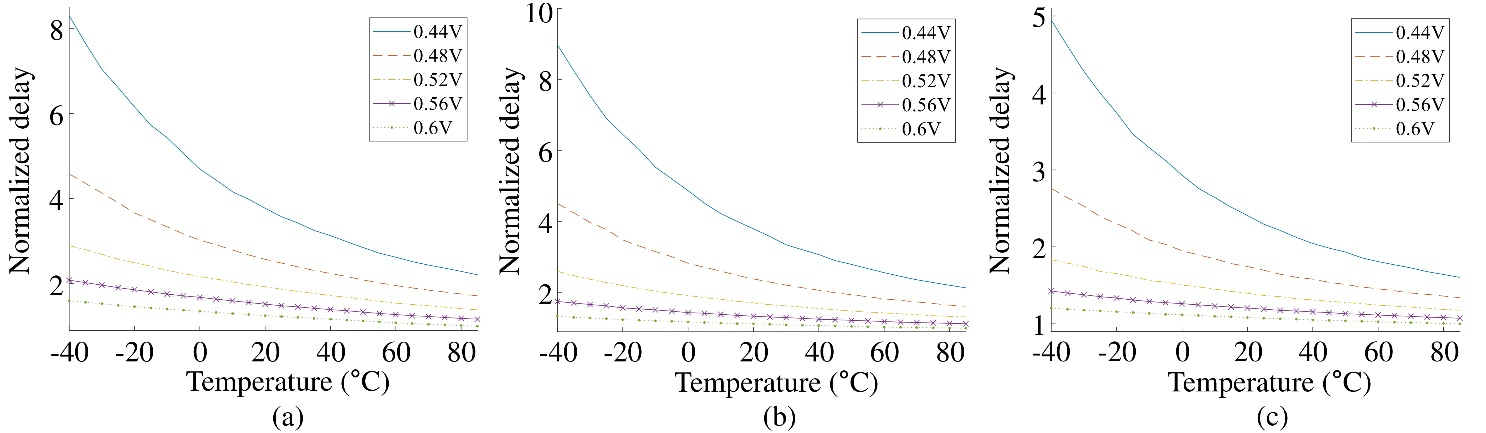## IV. ADVANCED TEI-VS FOR ULV-SRAM

The previous section clearly showed that TEI-VS can be used in ULV-SRAM. By applying TEI-VS, as the operating temperature of the ULV-SRAM increases, the supply voltage can be lowered to reduce power consumption. However, based on the fact that unlike logic circuits, ULV-SRAM is particularly vulnerable to random process variations, and this vulnerability is getting worse as ULV-SRAM goes to high temperature and low voltage, serious problems may arise if TEI-VS is applied to ULV-SRAM in the same way that TEI-VS is applied to logic circuits. Therefore, more sophisticated control is required to stably apply TEI-VS to ULV-SRAM. To this end, we propose the advanced TEI-VS for ULV-SRAM (TEI-VSUS for short) utilizing SNM as a measure of stability.

Algorithm 1 shows the pseudo-code of the proposed TEI-VSUS that lowers supply voltage while maintaining the operating speed and ensuring the minimum SNM value in the entire operating temperature range ($T_{min}\leq T\leq T_{\max }$) at the baseline supply. In the algorithm, the control resolution is $N$, so the temperature resolution to apply the TEI-VSUS is $\left(T_{max}-T_{min}\right)/N$. $T_{n}$ is set in ascending order by this temperature resolution (cf. line 2 in Algorithm 1). For a given target frequency $f_{target}$, the corresponding baseline voltage level of the ULV-SRAM is set to $V_{base}$. In the algorithm, we also introduce a design parameter $\delta$ as a percentage to control the minimum allowable SNM in the algorithm.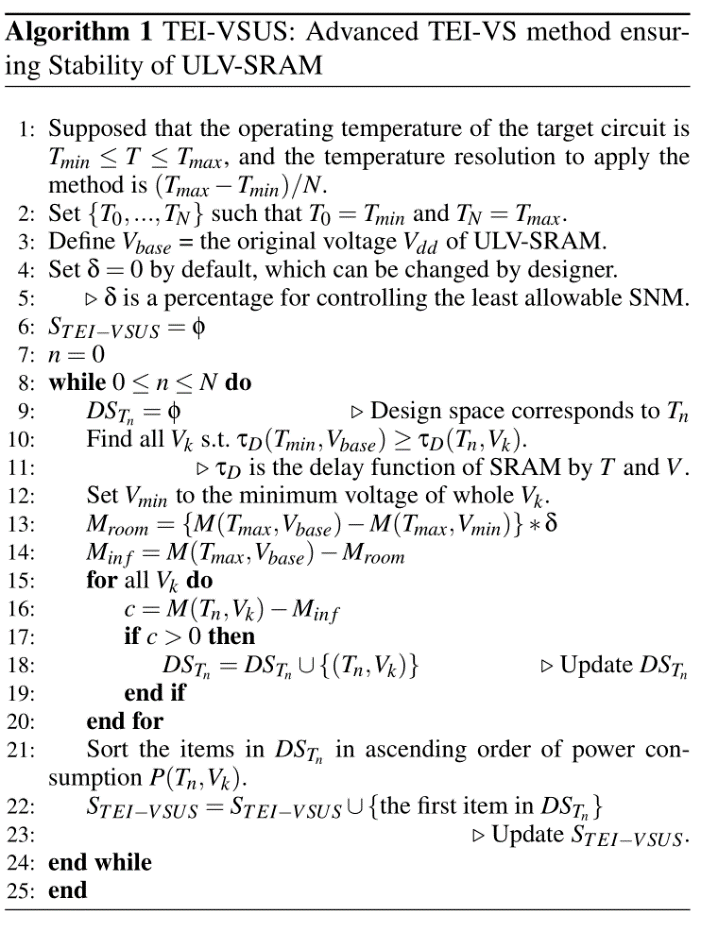Meanwhile, in Algorithm 1, the design space at the specific temperature $T_{n}$ is represented by $DS_{{T_{n}}}$. When some pairs of temperature and voltage are included in $DS_{{T_{n}}}$, the pairs will be sorted in ascending order of power consumption. In addition, for a certain temperature $T_{i}$ and voltage $V_{j}$, the corresponding delay and SNM value of the ULV-SRAM are $\tau _{D}\left(T_{i},V_{j}\right)$ and $M\left(T_{i},V_{j}\right)$, respectively.

Then, in Algorithm 1, we first find all $V_{k}$ that meets $\tau _{D}\left(T_{\min },V_{base}\right)\geq \tau _{D}\left(T_{n},V_{k}\right)\,,$ where $0\leq n\leq N\,.$ For reference, $V_{k}$ may be adjusted from the discrete voltage levels by a DC-DC converter that are within range of ensuring the operating of SRAM. From the set of $V_{k}$’s, we find $V_{min}$ (cf. line 12 in Algorithm 1). Next, we check the SNM. When setting $\delta$ to 0 by default, $M_{room}$ is 0, but $M_{inf}$ is the SNM value at $V_{base}$ and $T_{max}$. And this $M_{inf}$ represents the minimum SNM value over the all operating temperature range, because the higher the temperature, the smaller the SNM. We then update $DS_{{T_{n}}}$ so as to include $\left(T_{n},V_{k}\right)$ satisfying $M\left(T_{n},V_{k}\right)>M_{inf}$.

Finally, after sorting $DS_{{T_{n}}}$ in an ascending order of power consumption $P\left(T_{n},V_{k}\right)$ (cf. line 21 in Algorithm 1), we update $S_{TEI-VSUS}$ to take the first item in $DS_{{T_{n}}}$. As a result, the item $\left(T_{s},V_{s}\right)$ included in $S_{TEI-VSUS}$ means that when a given temperature reaches $T_{s}$, the supply is changed to $V_{s}$ to maintain $f_{target}$ and SVM of the SRAM and drive the SRAM with the lowest power consumption.

Using the proposed TEI-VSUS, it is possible to derive power gain at low $V_{dd}$ and ensure stability without performance degradation. These gains may be sufficient to demonstrate the advantages of the TEI-ULP techniques. However, in addition to modifying the bitcell structure, there exist additional techniques (e.g., error-correcting codes, interleaving schemes) that can improve the stability of SRAM [36,37]. Furthermore, SRAM intended for error-resilient applications may not need to set such strict limits on stability . The use of these assistive techniques would be less or less likely to require the high stability limiting level of the proposed TEI-VSUS. Therefore, we leave room for relaxation of the limitation on stability by allowing ${\delta}$ to be variable on the algorithm to achieve efficient voltage scaling using TEI-VSUS, not only with various SRAM structures but also using the assist schemes. More precisely, ${\delta}$ can be set between 0 and 1 for gradual control of the minimum allowable SNM. Smaller ${\delta}$ is more conservative in stability, but less effective at saving power in voltage scaling. $M_{room}$ is the actual control value over how much to allow the minimum SNM value determined by ${\delta}$.

## V. EXPERIMENTAL WORK

We conducted our research with the aim of ultimately incorporating the proposed method into the entire SoC, thus in this experimental work, targeting the application of the developed technique to an SoC presented in , which had proved the TEI-ULP techniques in the real chip. To this end, we performed all experiments with the same 28 nm FD-SOI as the semiconductor technology of the SoC. Then, we first designed the existing ULV-SRAM model mentioned in section 2 using Cadence Virtuoso based on 28 nm FD-SOI PDK. As a result, as mentioned above, the stability of the SRAM cell was checked through SNM in Fig. 5 and 6, and the TEI phenomenon was observed in ULV-SRAM through the simulation results shown in Fig. 7 and 8. Next, to validate the efficacy of the proposed TEI-VSUS, we used the designed ULV-SRAM, set the operating temperature range from from $-40$ to 80$\mathrm{℃}$ with resolution of 10$\mathrm{℃}$, and set the voltage control in units of 10 mV.

When applying TEI-VSUS with $\delta =0$ to the ULV-SRAMs, Table 1 provides the resulting maximum voltage scaling and the corresponding temperature range for each SRAM model. Even though the delta is set to 0, that is, the most conservative setting for stability, from the table results, we can confirm that TEI-VSUS can effectively lower the supply voltage. More specifically, as shown in the table, setting four different reference voltages allows voltage scaling up to 30 mV over a specific temperature range. For example, the 8T model with $V_{base}$ of 0.56 V can scale down to 0.53 V when the temperature is $-$2 to 10 $\mathrm{℃}$, and the 10T model with $V_{base}$ of 0.52V can scale down to 0.50V when the temperature is $-$14 to 31$\mathrm{℃}$. Meanwhile, in 28 nm FD-SOI technology, the trend of voltage scaling due to delay and stability is opposite. In other words, the temperature-dependent delay condition allows the voltage to be reduced by a larger magnitude at higher temperatures, but the temperature-dependent stability issue favors voltage scaling at lower temperatures. Therefore, the degree of voltage scaling according to the temperature change becomes a convex function.

##### Table 1. Minimum supply voltage and corresponding temperature range when applying the proposed TEI-VSUS with $\delta =0$
 Model 8T 10T 12T $V_{base}$ Supply Temp. Supply Temp. Supply Temp. $V_{dd}=0.60\mathrm{V}$ 0.57 V 1 to 15℃ 0.57 V 7 to 20℃ 0.58 V -13 to 25℃ $V_{dd}=0.56\mathrm{V}$ 0.53 V -2 to 10℃ 0.53 V 3 to 10℃ 0.54 V -15 to 15℃ $V_{dd}=0.52\mathrm{V}$ 0.50 V -16 to 31℃ 0.50 V -14 to 31℃ 0.50 V -17 to -3℃ $V_{dd}=0.48\mathrm{V}$ 0.46 V -18 to 20℃ 0.46 V -16 to 21℃ 0.47 V -29 to 38℃

We then performed experimental work with various ${\delta}$ values. In this experiment, we fixed $V_{base}$ to 0.6 V. Fig. 9 shows allowable $V_{dd}$ lowered by TEI-VSUS with different ${\delta}$’s. Although the effect of delta on the allowable voltage at low temperatures is weak, the scalable voltage varies significantly with the ${\delta}$ as the temperature increases. In particular, when ${\delta}$ is 1 (i.e., 100%), TEI-VSUS perfectly matches the conventional TEI-VS. In other words, it can be said that the temperature range in which the TEI phenomenon can be fully utilized is determined by ${\delta}$. More precisely, as seen in Fig. 9(a), minimum scalable voltage in 8T model are altered to $0.57\mathrm{V}$, $0.56\mathrm{V}$, $0.55\mathrm{V}$, $0.51\mathrm{V}$ for each delta $0\%$, $20\%$, $40\%$, $100\%$, respectively. Fig. 9(b) and (c) also show similar results. Namely, the more aggressive voltage scaling is available in high temperature with high values of ${\delta}$.

Next, according to the simulation results in Fig. 9, power consumption by temperature was measured to estimate the energy efficiency when TEI-VSUS is used as ULV-SRAM. Fig. 10-12 show the power saving rates for three operations (i.e., read, write, and hold operations) when using TEI-VSUS according to the different ${\delta}$’s. In the figures, we choose the representative delta value is 0, 0.2, 0.4 and 1 (i.e. $0\%$, $20\%$, $40\%$ and $100\%$). The power saving rate is derived from $\frac{\left(P_{\text{base}}-P_{TEI-VS\mathrm{US}}\right)}{P_{\text{base}}}\mathrm{*}100\left(\%\right)$, where $P_{base}$ and $P_{TEI-VSUS}$ are power consumption on the baseline voltage $V_{base}$ and the scaled voltage by TEI-VSUS, respectively.

In Fig. 10-12, it can be seen that even under the most conservative condition (i.e., $\delta =0$), power saving can be achievable in all the operations and models between $-20$ and $60\mathrm{℃}$. Taking the 10T model as an example in a more detail, as shown in Fig. 10(b) and 11(b), in the 10T model, when the supply voltage is scaled from 0.6 to 0.57 V at 10$\mathrm{℃}$ (cf. Fig. 9), 12.3% and 18.2% power saving rates are reported for the write and read operation, respectively, without any performance penalty. For the hold operation of 10T, which accounts for most of the static power, as shown in Fig. 12(b), the hold power saving rate of the 10T model is 18.2% at 10$\mathrm{℃}$. The maximum power saving efficiency of the 10T model is 20$\mathrm{℃}$ for write operation and 10 $\mathrm{℃}$ for read and hold operation. In addition, power saving rate of the write operation increases from $4.2\%$ up to $12.3\%$ when temperature is below $20\mathrm{℃}$, after then gradually decreases until $4.1\%$. Other operations tend to be similar to write operation, but the difference is the value of the peak temperature and the corresponding power saving rate. which are $10\mathrm{℃}$, $18.2\%$ for read operation and $10\mathrm{℃}$, $16.8\%$ for hold operation.

Meanwhile, when ${\delta}$ is increased, the power saving effect of TEI-VSUS increases. For example, comparing the case where ${\delta}$ is 0 and 0.2 for each model through Fig. 10-12, it can be observed that the maximum power saving rate is increased and the corresponding temperature range is also increased. To explain this in more detail based on the 10T model, when the voltage scaling can go down to 0.56 V at 30$\mathrm{℃}$, the power saving rates are 16.0, 21.9, and 20.8% for the write, read, and hold operations, respectively. When ${\delta}$ is 0.4, when the supply voltage can be reduced to 0.55 V at 40$\mathrm{℃}$, the power saving rate becomes 19.4, 25.2, and 24.8% for the write, read and hold operations, respectively. Even when ${\delta}$ is set to be 1, the minimum voltage scaling is 0.51 V at 80$\mathrm{℃}$, and the power saving rate increases simultaneously to 27.6% for the write, 30.2% for the read, and 34.3% for the hold operation. Therefore, we can confirm that the higher ${\delta}$, the lower the scaling voltage is only available in the high temperature range, and it also increases the temperature range where the TEI-VSUS has the highest efficiency. The 8T and 12T models also show a similar trend to the results of the 10T model.

##### Fig. 9. Minimum operating voltage in bit-cell structure when TEI-VS is applied to (a) 8T; (b) 10T; (c) 12T models.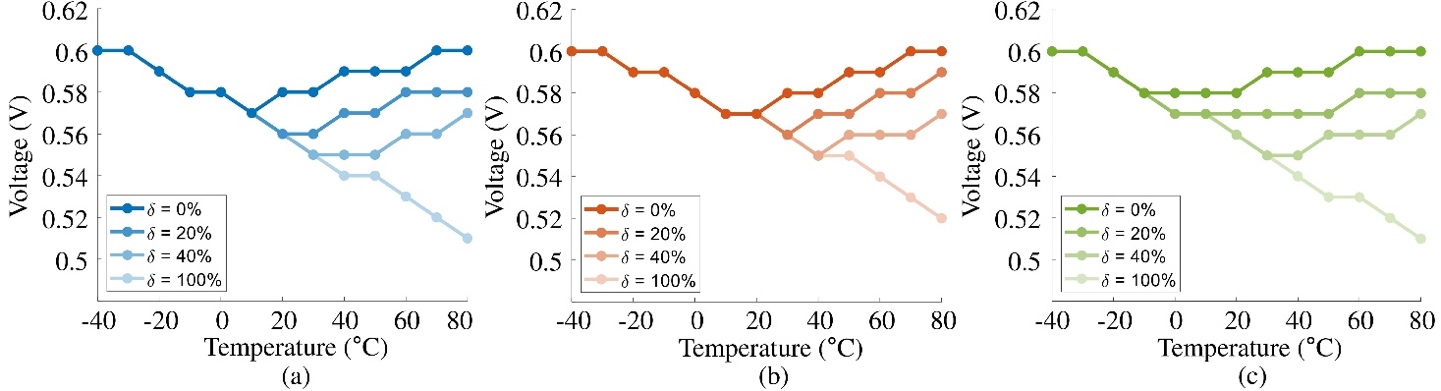##### Fig. 10. Power saving of (a) 8T; (b) 10T; (c) 12T models with TEI-VS at the Write operation.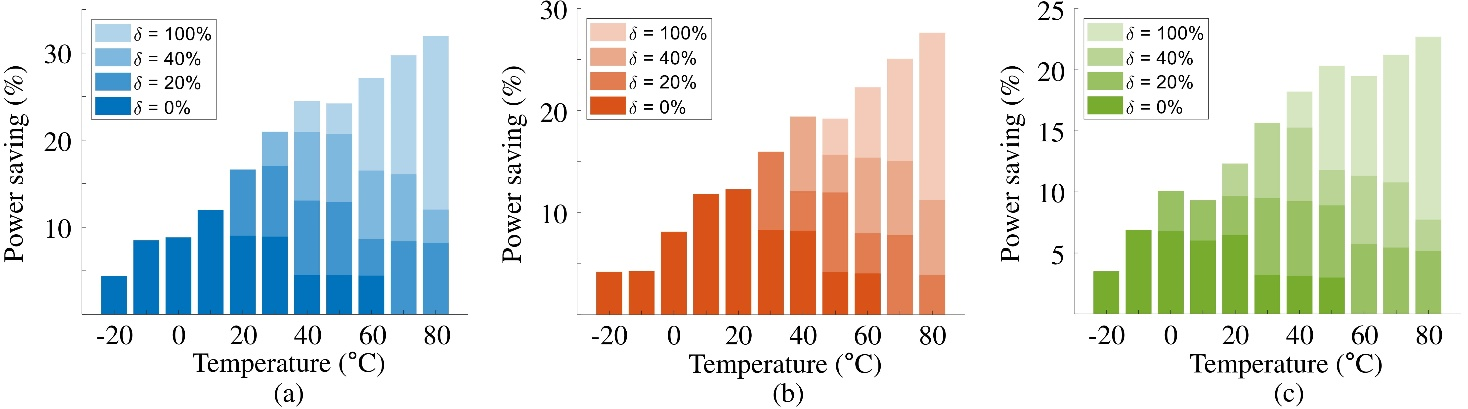##### Fig. 11. Power saving of (a) 8T; (b) 10T; (c) 12T models with TEI-VS at the Read operation.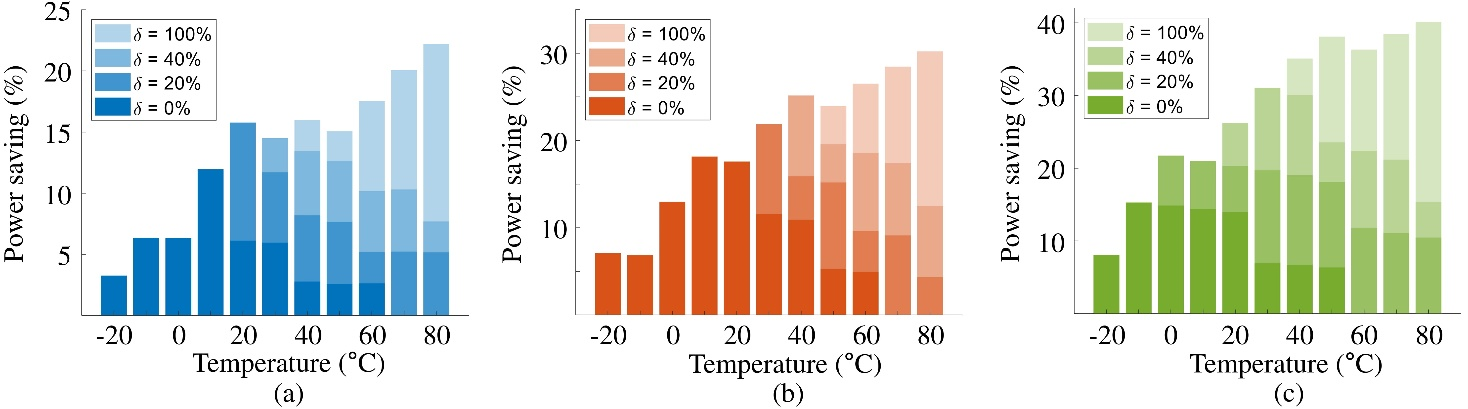##### Fig. 12. Power saving of (a) 8T; (b) 10T; (c) 12T models with TEI-VS at the Hold operation.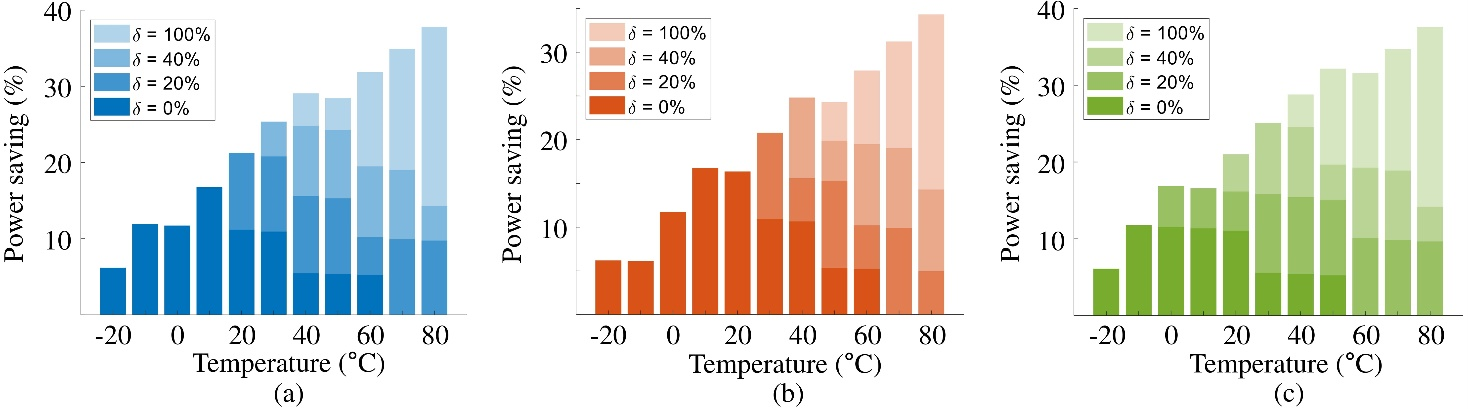Finally, we show that it is possible to utilize TEI-VS technique in ULV-SRAM, while demonstrating that power savings can be performed without loss of speed and loss of minimum SNM through the proposed TEI-VSUS. In addition, an adjustable ${\delta}$ is introduced to make the TEI-VSUS algorithm more flexible and generally applicable, and a detailed experiment is conducted for this purpose. In particular, we clearly revealed how the power saving rate and its tendency change depending on the ${\delta}$ change. This allows SoC designers to apply other assist techniques to compensate for the decrease in stability due to voltage drop, increasing ${\delta}$ to lower the minimum SNM value but making more aggressive voltage scaling.

## V. CONCLUSIONS

In this paper, we have revealed for the first time that the TEI phenomenon occurs in the existing ULV-SRAM. Furthermore, considering the stability problem of SRAM that makes it difficult to apply the existing TEI-VS to SRAM, we have proposed TEI-VSUS, an advanced TEI-VS technology that solves this problem. Subsequently, TEI-VSUS has been verified in ULV-SRAM through simulation, and the power saving rate for each operation and SRAM model has been obtained. In addition, an method to increase the power saving effect of TEI-VSUS has been proposed by relaxing the restrictions on stability so that the proposed technique can be used in a wider environment. The effect of the proposed method has also been verified through SRAM model simulations based on the 28 nm FD-SOI technology node.

## ACKNOWLEDGMENTS

This work was partially supported by the Chung-Ang University Graduate Research Scholarship in 2020, and partially supported by the National R&D Program through the National Research Foundation of Korea (NRF) funded by Ministry of Science and ICT (2021M3H2A1038042)

## References

1
Conti F., Schilling R., Schiavone P.D., Pullini A., Rossi D., Gürkaynak F.K., Muehlberghuber M., Gautschi M., Loi I., Haugou G., Mangard S., Benini L., 2017, An IoT endpoint system-on-chip for secure and energy-efficient near-sensor analytics, IEEE Trans. on Circuits and Systems I: Regular Papers, Vol. 64, pp. 2481-24942
Magno M., Aoudia F.A., Gautier M., Berder O., Benini L. WULoRa., 2017, an energy efficient IoT end-node for energy harvesting and heterogeneous communication, Proc. of Int. Conf. on Design, Automation & Test in Europe, pp. 1528-15333
Fayyazi A., Ansari M., Kamal M., Afzali-Kusha A., Pedram M., 2018, An ultra low-power memristive neuromorphic circuit for internet of things smart sensors, IEEE Internet of Things Journal, Vol. 5, pp. 1011-10224
Ciccia S., Giordanengo G., Vecchi G., 2019, Energy Efficiency in IoT Networks: Integration of Reconfigurable Antennas in Ultra Low-Power Radio Platforms Based on System-on-Chip, IEEE Internet of Things Journal, Vol. 6, pp. 6800-68105
Han K., Lee S., Lee J.j., Lee W., Pedram M., 2019, TIP : A Temperature Effect Inversion-Aware Ultra-Low Power System-on-Chip Platform, 2019 IEEE/ACM International Symposium on Low Power Electronics and Design, pp. 1-66
Alioto M., 2012, Ultra-low power VLSI circuit design demystified and explained: A tutorial, IEEE Transactions on Circuits and Systems I: Regular Papers, Vol. 59, pp. 3-297
Rossi D., Pullini A., Loi I., Gautschi M., Gürkaynak F.K., Teman A., Constantin J., Burg A., Miro-Panades I., Beigne E., Clermidy F., Abouzeid F., Flatresse P., Benini L., 2016, 193 MOPS/mW @ 162 MOPS, 0.32V to 1.15V voltage range multi-core accelerator for energy efficient parallel and sequential digital processing, Proc. of Symp. on Low-Power and High-Speed Chips and Systems8
Gautschi M., Schiavone P.D., Member S., Traber A., Loi I., Pullini A., Rossi D., Flamand E., Gürkaynak F.K., Benini L., 2017, Near-threshold RISC-V core with DSP extensions for scalable IoT endpoint devices, IEEE Trans. on Very Large Scale Integration Systems, Vol. 25, pp. 2700-27139
Karnik T., Kurian D., Aseron P., Dorrance R., Alpman E., Nicoara A., Popov R., Azarenkov L., Moiseev M., Zhao L., Ghosh S., Misoczki R., Gupta A., M A., Muthukumar S., Bhandari S., Satish Y., Jain K., Flory R., Kanthapanit C., Quijano E., Jackson B., Luo H., Kim S., Vaidya V., Elsherbini A., Liu R., Sheikh F., Tickoo O., Klotchkov I., Sastry M., Sun S., Bhartiya M., Srinivasan A., Hoskote Y., Wang H., De V., 2018, A cm-scale self-powered intelligent and secure IoT edge mote featuring an ultra-low-power SoC in 14 nm tri-gate CMOS, Proc. of Int. Solid-State Circuits Conference Digest of Technical Papers, pp. 46-4810
Pu Y., Shi C., Samson G., Park D., Beraha R., Newham A., Lin M., Rangan V., Chatha K., Butterfield D., Attar R., 2018, A 9-mm2 ultra-low-power highly integrated 28-nm CMOS SoC for internet of things, IEEE Journal of Solid-State Circuits, Vol. 53, pp. 936-94811
STMicroelectronics. , STM32L151C6: ultra-low-power ARM Cortex-M3 MCU with 32 Kbytes flash, 32 MHz CPU, USB, https://www.st.com/en/microcontrollers/stm32l151c6.html. Accessed 15 Feb. 2022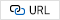12
Maxim integrated. , MAX32626: ultra-low power, high-performance ARM Cortex-M4 with FPU-based microcontroller for wearables, http://www.maximintegrated.com/en/products/microcontrollers/MAX32626.html. Accessed 15 Feb. 202213
NXP. , K32W0x MCUs for wireless IoT applications, https://www.nxp.com/docs/en/fact-sheet/K32W0XFS.pdf. Accessed 15 Feb. 202214
Lee W., Wang Y., Cui T., Nazarian S., Pedram M., 2015-October, Dynamic thermal management for FinFET-based circuits exploiting the temperature effect inversion phenomenon, Proceedings of the International Symposium on Low Power Electronics and Design 2015, pp. 105-11015
Cai E., Marculescu D., TEI-Turbo: Temperature effect inversion-aware turbo boost for finfet-based multi-core systems, 2015 IEEE/ACM International Conference on Computer-Aided Design, ICCAD 2015 2016, pp. 500-50716
Rossi D., Pullini A., Loi I., Gautschi M., Gürkaynak F.K., Bartolini A., Flatresse P., Benini L., 2016, A 60 gops/w,− 1.8 v to 0.9 v body bias ulp cluster in 28 nm utbb fd-soi technology, Solid-State Electronics, Vol. 117, pp. 170-18417
Lee W., Han K., Wang Y., Cui T., Nazarian S., Pedram M., 2017, TEI-power: Temperature effect inversion-aware dynamic thermal management, ACM Transactions on Design Automation of Electronic Systems, Vol. 2218
Park J., Cha H., 2017, Aggressive voltage and temperature control for power saving in mobile application processors, IEEE Trans. on Mobile Computing, Vol. 17, pp. 1233-124619
Han K., Lee J.J., Lee J., Lee W., Pedram M., 2018, TEI-NoC: Optimizing ultralow power NoCs exploiting the temperature effect inversion, IEEE Transactions on Computer-Aided Design of Integrated Circuits and Systems, Vol. 37, pp. 458-47120
2019, TEI-ULP: Exploiting Body Biasing to Improve the TEI-Aware Ultralow Power Methods, IEEE Transactions on Computer-Aided Design of Integrated Circuits and Systems, Vol. 38, pp. 1758-177021
Han K., Lee S., Oh K.I., Bae Y., Jang H., Lee J.J., Lee W., Pedram M., 2021, Developing TEI-Aware Ultralow-Power SoC Platforms for IoT End Nodes, IEEE Internet of Things Journal, Vol. 8, pp. 4642-465622
Chien Y.C., Wang J.S., 2018, A 0.2 v 32-Kb 10T SRAM with 41 nW Standby Power for IoT Applications, IEEE Transactions on Circuits and Systems I: Regular Papers, Vol. 65, pp. 2443-245423
Sarfraz K., He J., Chan M., 2017, A 140-mV Variation-Tolerant Deep Sub-Threshold SRAM in 65-nm CMOS, IEEE Journal of Solid-State Circuits, Vol. 52, pp. 2215-222024
Verma N., Chandrakasan A.P., 2008, A 256 kb 65 nm 8T Subthreshold SRAM Employing Sense-Amplifier Redundancy, IEEE Journal of Solid-State Circuits, Vol. 43, pp. 141-14925
Chang I.J., Kim J.J., Park S.P., Roy K., 2009, A 32 kb 10T sub-threshold sram array with bit-interleaving and differential read scheme in 90 nm CMOS, IEEE Journal of Solid-State Circuits, Vol. 44, pp. 650-65826
Chiu Y.W., Hu Y.H., Tu M.H., Zhao J.K., Chu Y.H., Jou S.J., Chuang C.T., 2014, 40 Nm Bit-Interleaving 12T Subthreshold Sram With Data-Aware Write-Assist, IEEE Transactions on Circuits and Systems I: Regular Papers, Vol. 61, pp. 2578-258527
Kim D., Chandra V., Aitken R., Blaauw D., Sylvester D., 2011, Variation-aware static and dynamic writability analysis for voltage-scaled bit-interleaved 8-T SRAMs, Proceedings of the International Symposium on Low Power Electronics and Design, pp. 145-15028
Maiz J., Hareland S., Zhang K., Armstrong P., 2003, Characterization of Multi-bit Soft Error events in advanced SRAMs, Technical Digest - International Electron Devices Meeting, pp. 519-52229
Qazi M., Sinangil M.E., Chandrakasan A.P., 2011, Challenges and directions for low-voltage SRAM, IEEE Design and Test of Computers, Vol. 28, pp. 32-4330
Zhai B., Hanson S., Blaauw D., Sylvester D., 2008, A Variation-Tolerant Sub-200 mV 6-T Subthreshold SRAM, IEEE Journal of Solid-State Circuits, Vol. 43, pp. 2338-234831
Seevinck E., List F.J., Lohstroh J., 1987, Static-noise margin analysis of MOS SRAM cells, IEEE Journal of Solid-State Circuits, Vol. 22, pp. 748-75432
Kim T., Liu J., Keane J., Kim C.H., 2008, A 0.2 V, 480 kb Subthreshold SRAM With 1 k Cells Per Bitline for Ultra-Low-Voltage Computing, IEEE Journal of Solid-State Circuits, Vol. 43, pp. 518-52933
Islam A., Hasan M., 2012, A technique to mitigate impact of process, voltage and temperature variations on design metrics of SRAM Cell, Microelectronics Reliability, Vol. 52, pp. 405-41134
Hamdioui S., 2001, Testing multi-port memories: Theory and practice"35
Slayman C. W., Sept. 2005, Cache and memory error detection, correction, and reduction techniques for terrestrial servers and workstations, in~IEEE Transactions on Device and Materials Reliability, Vol. 5, No. 3, pp. 397-40436
Baeg S., Wen S., Wong R., Aug. 2009, SRAM Interleaving Distance Selection With a Soft Error Failure Model, in~IEEE Transactions on Nuclear Science, Vol. 56, No. 4, pp. 2111-211837
Frustaci F., Khayatzadeh M., Blaauw D., Sylvester D., Alioto M., May 2015, SRAM for Error-Tolerant Applications With Dynamic Energy-Quality Management in 28 nm CMOS, in IEEE Journal of Solid-State Circuits, Vol. 50, No. 5, pp. 1310-1323Seung-Yeong Lee received the B.S. degree from Chung-Ang University, Seoul, South Korea, in 2020, where he is currently pursuing the M.S. degree in electrical and electronics engineering. He is a beneficiary student of the High-Potential Individuals Global Training Program. His research interest includes low power design, SoC architecture and embedded system.

Jae-Hyoung Lee received the B.S. degree from the Myoungji University, Yong-In, South Korea, in 2020, and is in Chung-Ang University, where he is currently pursuing the M.S. degree in electrical and electronics engineering. He is a beneficiary student of the High-Potential Individuals Global Training Program His research interest includes low power design, SoC architecture and embedded system.

Woojoo Lee received his B.S. (2007) in electrical engineering from Seoul National University, Seoul, Korea, and his M.S. (2010) and Ph.D. (2015) degrees in electrical engineering from University of Southern California, Los Angeles, CA. He was with Electronics and Telecommunications Research Institute (2015-2016) as a senior researcher in SoC Design Research Group, Department of Electrical Engineering at Myongji University (2017-2018) as an assistant professor. He is currently an associate professor with the School of Electrical & Electronics Engineering, Chung-Ang University, Seoul, Korea. His research interest includes ultra-low power VLSI and SoC designs, embedded system designs, and system-level power and thermal management.

Younghyun Kim is currently an Assistant Professor of Electrical and Computer Engineering at the University of Wisconsin-Madison, Madison, WI, USA. His research interests include energy-efficient computing, machine learning at the edge, and cyber-physical systems. Kim received a Ph.D. degree in electrical engineering and computer science from Seoul National University in 2013. Before joining University of Wisconsin- Madison in 2016, he was a postdoc at Purdue University, West Lafayette, IN, USA. He is a member of IEEE and ACM.